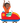# Slopes

#### What is slope?

Good question! Slope is a measure of how steep something is. It's what we're calculating when we divide vertical change by horizontal change of a line. Read below to learn more.

#### Common QuestionsSlope measures how steep something is. The steeper slope is always the bigger slope. So what does that really mean?

Imagine a roller coaster. At the beginning, usually the ride moves up slowly to terrify you before the drop.How scary it is depends on how steep the ramp up is, and we can measure that by how much vertical height you gain for every unit of horizontal distance. This is called the slope.

Try dragging the roller-coaster cart below to see how we calculate the slope.Notice we use two points to calculate the slope: the starting point and the point you drag the cart to. We divide the vertical change by the horizontal change.

Also notice that because we have a straight line, the slope never changes. As we drag along the ramp, the slope stays the same. For every 1 increase in horizontal distance, we get an increase of 1 in vertical distance.

Let's look at a slightly less steep ramp. Try dragging the cart!Here, the slope still stays the same no matter where you drag the cart. For every 1 increase in horizontal distance, we get an increase of in vertical distance.

And notice that to get an easier calculation without decimals, we want to drag our cart to a point without decimals in the distance, in this case .

Nice work! So remember,

#### Slope Formula

The slope of a line is a measure of how steep the line is. We calculate it with two easy steps:

1. Find two points. Let's call them Point 1 with coordinates and Point 2 with coordinates .
2. Divide the change in by the change in :
Notice the color coding - make sure that the first and values in the subtractions are from the same point.